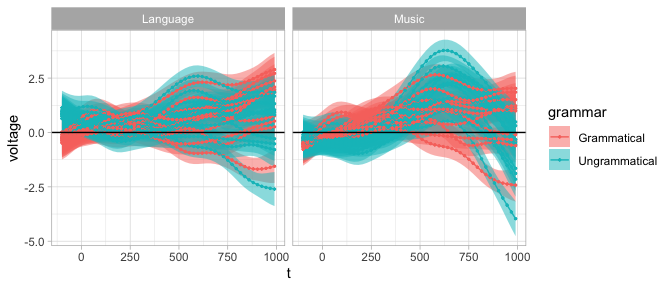# Get started

library(tidygam)
library(mgcv)
#> This is mgcv 1.8-40. For overview type 'help("mgcv-package")'.
library(dplyr)
#>
#> Attaching package: 'dplyr'
#> The following object is masked from 'package:nlme':
#>
#>     collapse
#> The following objects are masked from 'package:stats':
#>
#>     filter, lag
#> The following objects are masked from 'package:base':
#>
#>     intersect, setdiff, setequal, union
library(ggplot2)
theme_set(theme_light())

# Overview

The tidymv package offers two main user-oriented functions:

• predict_gam(): returns predictions of the outcome variable based on the predictors in the GAM model. The user can specify specific values for any predictor, and exclude model terms.

• get_difference(): returns the difference between two smooths and those intervals along the smooth that do not include 0.

The output of these function can then be plotted with plot(), through the methods plot.tidygam() and plot.tidygam.diff().

# Basic model prediction

We will use the gest data table, available in tidygam. The table consists of counts of gestures performed by infants of three cultural backgrounds who participating in a longitudinal study (see ?gest for details and references).

data("gest")
gest
#> # A tibble: 540 × 5
#>    dyad  background months gesture count
#>    <fct> <fct>       <dbl> <fct>   <dbl>
#>  1 b01   Bengali        10 ho_gv       0
#>  2 b01   Bengali        10 point       0
#>  3 b01   Bengali        10 reach       5
#>  4 b01   Bengali        11 ho_gv       0
#>  5 b01   Bengali        11 point       1
#>  6 b01   Bengali        11 reach       8
#>  7 b01   Bengali        12 ho_gv       3
#>  8 b01   Bengali        12 point       0
#>  9 b01   Bengali        12 reach       0
#> 10 b02   Bengali        10 ho_gv       1
#> # … with 530 more rows

The following GAM models the overall trend in number of gestures from 10 to 12 months of age.

gs <- gam(
count ~ s(months, k = 3),
data = gest,
family = poisson
)

summary(gs)
#>
#> Family: poisson
#>
#> Formula:
#> count ~ s(months, k = 3)
#>
#> Parametric coefficients:
#>             Estimate Std. Error z value Pr(>|z|)
#> (Intercept)  1.27491    0.02361   53.99   <2e-16 ***
#> ---
#> Signif. codes:  0 '***' 0.001 '**' 0.01 '*' 0.05 '.' 0.1 ' ' 1
#>
#> Approximate significance of smooth terms:
#>             edf Ref.df Chi.sq p-value
#> s(months) 1.861  1.981  248.9  <2e-16 ***
#> ---
#> Signif. codes:  0 '***' 0.001 '**' 0.01 '*' 0.05 '.' 0.1 ' ' 1
#>
#> R-sq.(adj) =  0.0372   Deviance explained = 6.61%
#> UBRE = 6.1921  Scale est. = 1         n = 540

Now we can obtain the predicted counts with predict_gam().

gs_pred <- predict_gam(gs)
gs_pred
#> # A tibble: 11 × 5
#>    months count     se lower_ci upper_ci
#>     <dbl> <dbl>  <dbl>    <dbl>    <dbl>
#>  1   10   0.768 0.0498    0.670    0.865
#>  2   10.2 0.896 0.0396    0.818    0.973
#>  3   10.4 1.02  0.0340    0.954    1.09
#>  4   10.6 1.14  0.0334    1.07     1.21
#>  5   10.8 1.25  0.0352    1.18     1.32
#>  6   11   1.35  0.0363    1.28     1.42
#>  7   11.2 1.44  0.0346    1.37     1.51
#>  8   11.4 1.51  0.0308    1.45     1.58
#>  9   11.6 1.58  0.0269    1.53     1.64
#> 10   11.8 1.65  0.0265    1.59     1.70
#> 11   12   1.71  0.0315    1.64     1.77

# Plot predicted values

predict_gam() returns an object of class tidygam, which can be plotted with plot().

The user has to specify the “series” used as the x-axis. The outcome variable is automatically selected for the y-axis.

gs_pred %>%
plot(series = "months")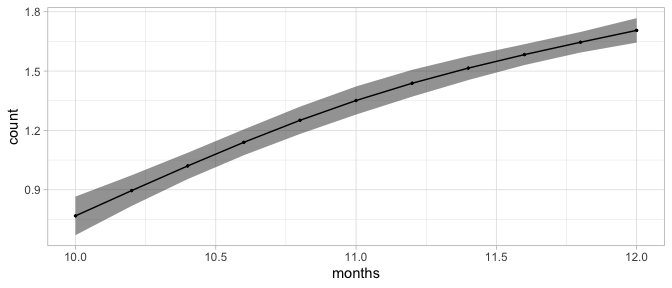Since the gs model used a log-link function, the output of predict_gam() is in log-odds, rather than in counts.

We can convert the log-odds to counts by exponentiating them. The tran_fun argument allows the user to specify a function to transform the predicted outcome values with.

predict_gam(gs, tran_fun = exp) %>%
plot(series = "months")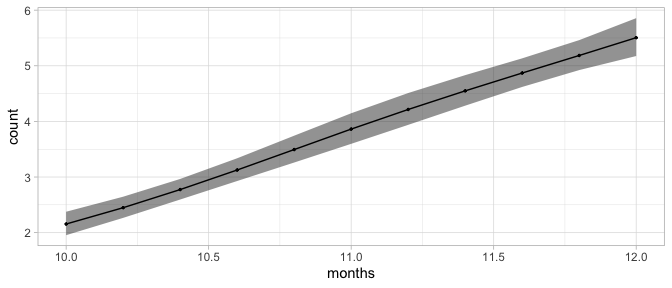# Models with by-variables

Smooths can be fitted to different levels of a factor using so-called by-variables, specified within the smooth function s() with the by argument.

In this model, we fit a smooth along months for each background in the data.

gs_by <- gam(
count ~ s(months, by = background, k = 3),
data = gest,
family = poisson
)

summary(gs_by)
#>
#> Family: poisson
#>
#> Formula:
#> count ~ s(months, by = background, k = 3)
#>
#> Parametric coefficients:
#>             Estimate Std. Error z value Pr(>|z|)
#> (Intercept)  1.26733    0.02376   53.34   <2e-16 ***
#> ---
#> Signif. codes:  0 '***' 0.001 '**' 0.01 '*' 0.05 '.' 0.1 ' ' 1
#>
#> Approximate significance of smooth terms:
#>                               edf Ref.df Chi.sq p-value
#> s(months):backgroundBengali 1.941  1.996  98.34  <2e-16 ***
#> s(months):backgroundChinese 1.015  1.029 168.53  <2e-16 ***
#> s(months):backgroundEnglish 1.000  1.000  39.39  <2e-16 ***
#> ---
#> Signif. codes:  0 '***' 0.001 '**' 0.01 '*' 0.05 '.' 0.1 ' ' 1
#>
#> R-sq.(adj) =   0.04   Deviance explained = 7.38%
#> UBRE = 6.1412  Scale est. = 1         n = 540

The predictor for comparison is selected with the comparison argument in plot().

gs_by %>%
predict_gam(length_out = 20, series = "months", tran_fun = exp) %>%
plot(comparison = "background")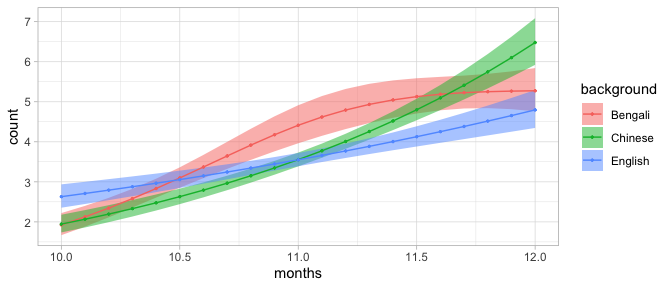Note that the output of plot() is a ggplot2 object, which can be modified using the ggplot2 machinery.

gs_by %>%
predict_gam(length_out = 20, series = "months", tran_fun = exp) %>%
plot(comparison = "background") +
scale_color_brewer(type = "qual") + scale_fill_brewer(type = "qual")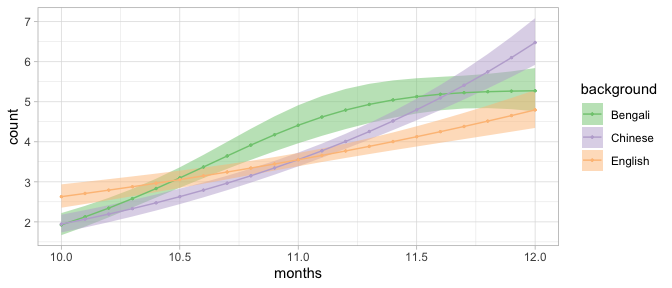Let’s try now a model with both gesture and background as by-variables.

gs_by_2 <- gam(
count ~ s(months, by = background, k = 3) +
s(months, by = gesture, k = 3),
data = gest,
family = poisson
)

summary(gs_by_2)
#>
#> Family: poisson
#>
#> Formula:
#> count ~ s(months, by = background, k = 3) + s(months, by = gesture,
#>     k = 3)
#>
#> Parametric coefficients:
#>             Estimate Std. Error z value Pr(>|z|)
#> (Intercept)  1.23541    0.02447    50.5   <2e-16 ***
#> ---
#> Signif. codes:  0 '***' 0.001 '**' 0.01 '*' 0.05 '.' 0.1 ' ' 1
#>
#> Approximate significance of smooth terms:
#>                                  edf   Ref.df  Chi.sq  p-value
#> s(months):backgroundBengali 1.905650 1.989138  16.600 0.000192 ***
#> s(months):backgroundChinese 1.001631 1.003219  14.594 0.000134 ***
#> s(months):backgroundEnglish 1.000270 1.000534   1.064 0.302390
#> s(months):gestureho_gv      1.737652 1.929211 111.567  < 2e-16 ***
#> s(months):gesturepoint      1.000045 1.000090  25.537  8.9e-07 ***
#> s(months):gesturereach      0.004305 0.008524   0.004 0.949548
#> ---
#> Signif. codes:  0 '***' 0.001 '**' 0.01 '*' 0.05 '.' 0.1 ' ' 1
#>
#> Rank: 12/13
#> R-sq.(adj) =  0.0714   Deviance explained = 10.4%
#> UBRE = 5.9192  Scale est. = 1         n = 540

Note that models like this one are conceptually equivalent to linear models without interactions between the by-variables.

This is clear when plotting the predictions: notice how the shapes of the smooths are very similar within each background, and they only differ in slope (this is the effect of including separate by-variables).

gs_by_2 %>%
predict_gam(length_out = 20, series = "months", tran_fun = exp) %>%
plot(comparison = "gesture") +
scale_color_brewer(type = "qual") + scale_fill_brewer(type = "qual") +
facet_grid(~ background)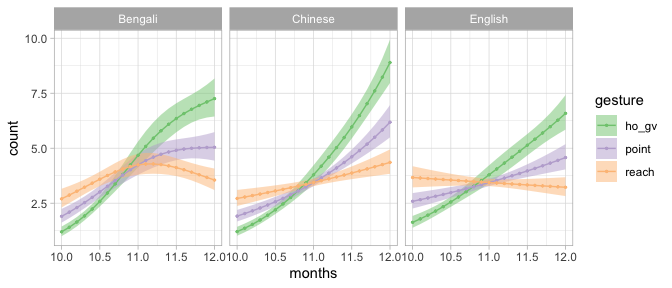The following section illustrates how to specify and plot models with the GAM equivalent of classical interactions (e.g. background * gesture).

# Models with factor interactions

Classical interactions between factors as usually obtained in linear models with the : syntax (e.g. background:gesture) are not possible in GAMs.

An alternative way to specify what are called interactions in generalised linear models is by creating a new factor which is the interaction of the two or more factors using the interaction() function, and include this “factor interaction” predictors as a by-variable.

gest <- gest %>%
mutate(back_gest = interaction(background, gesture))

gs_i <- gam(
count ~ s(months, by = back_gest, k = 3),
data = gest,
family = poisson
)

summary(gs_i)
#>
#> Family: poisson
#>
#> Formula:
#> count ~ s(months, by = back_gest, k = 3)
#>
#> Parametric coefficients:
#>             Estimate Std. Error z value Pr(>|z|)
#> (Intercept)  1.22513    0.02466   49.68   <2e-16 ***
#> ---
#> Signif. codes:  0 '***' 0.001 '**' 0.01 '*' 0.05 '.' 0.1 ' ' 1
#>
#> Approximate significance of smooth terms:
#>                                    edf Ref.df  Chi.sq  p-value
#> s(months):back_gestBengali.ho_gv 1.944  1.997 197.196  < 2e-16 ***
#> s(months):back_gestChinese.ho_gv 1.792  1.957 146.664  < 2e-16 ***
#> s(months):back_gestEnglish.ho_gv 1.001  1.002  36.608  < 2e-16 ***
#> s(months):back_gestBengali.point 1.904  1.991  13.941 0.000649 ***
#> s(months):back_gestChinese.point 1.032  1.063 104.711  < 2e-16 ***
#> s(months):back_gestEnglish.point 1.001  1.002  12.431 0.000425 ***
#> s(months):back_gestBengali.reach 1.753  1.939   3.005 0.172851
#> s(months):back_gestChinese.reach 1.667  1.889   2.295 0.218191
#> s(months):back_gestEnglish.reach 1.000  1.000   2.655 0.103233
#> ---
#> Signif. codes:  0 '***' 0.001 '**' 0.01 '*' 0.05 '.' 0.1 ' ' 1
#>
#> R-sq.(adj) =  0.074   Deviance explained = 11.5%
#> UBRE = 5.8613  Scale est. = 1         n = 540

When predicting values, the user can use the separate argument to specify factor-interaction variables in the model that can be split back into their individual components.

This gives greater flexibility when plotting.

predict_gam(
gs_i, tran_fun = exp,
separate = list(back_gest = c("background", "gesture"))
) %>%
plot(series = "months", comparison = "gesture") +
facet_grid(~ background)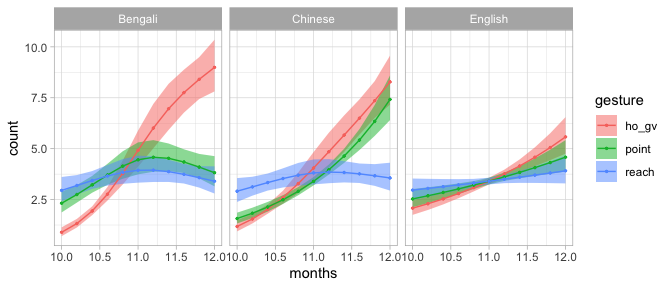# Models with factor smooth interactions (bs = "fs")

Factor smooth interactions are the GAM equivalent of random/group-level effects (intercepts and slopes).

Let’s work with the struct data, which contains event-related potentials measures of subjects listening to music and speech. For each type (music vs language), the stimuli were either “grammatical” or “ungrammatical” (i.e. the stimuli either respected structural rules or they did not).

This is a subset of the original data, including voltage values only for electrode 62.

data("struct")
struct
#> # A tibble: 4,400 × 7
#>        t electrode voltage subject stimulus.condition grammar.conditi… stim_gram
#>    <dbl>     <dbl>   <dbl> <fct>   <fct>              <fct>            <fct>
#>  1  -100        62 -0.315  03      Language           Grammatical      Language…
#>  2   -90        62 -0.320  03      Language           Grammatical      Language…
#>  3   -80        62 -0.297  03      Language           Grammatical      Language…
#>  4   -70        62 -0.628  03      Language           Grammatical      Language…
#>  5   -60        62 -1.05   03      Language           Grammatical      Language…
#>  6   -50        62 -0.734  03      Language           Grammatical      Language…
#>  7   -40        62  0.0544 03      Language           Grammatical      Language…
#>  8   -30        62  0.623  03      Language           Grammatical      Language…
#>  9   -20        62  1.05   03      Language           Grammatical      Language…
#> 10   -10        62  1.14   03      Language           Grammatical      Language…
#> # … with 4,390 more rows

Let’s fit the model with factor smooth interactions (bs = "fs").

struct <- struct %>%
mutate(stim_gram = interaction(stimulus.condition, grammar.condition))

st <- bam(
voltage ~
s(t, by = stim_gram, k = 5) +
s(t, subject, bs = "fs", m = 1),
data = struct
)

summary(st)
#>
#> Family: gaussian
#>
#> Formula:
#> voltage ~ s(t, by = stim_gram, k = 5) + s(t, subject, bs = "fs",
#>     m = 1)
#>
#> Parametric coefficients:
#>             Estimate Std. Error t value Pr(>|t|)
#> (Intercept)   0.5139     0.1816    2.83  0.00468 **
#> ---
#> Signif. codes:  0 '***' 0.001 '**' 0.01 '*' 0.05 '.' 0.1 ' ' 1
#>
#> Approximate significance of smooth terms:
#>                                         edf Ref.df      F p-value
#> s(t):stim_gramLanguage.Grammatical    2.777  3.187  3.403 0.02385 *
#> s(t):stim_gramMusic.Grammatical       2.607  3.009  4.182 0.00567 **
#> s(t):stim_gramLanguage.Ungrammatical  3.246  3.616  2.766 0.10796
#> s(t):stim_gramMusic.Ungrammatical     3.842  3.961 23.519 < 2e-16 ***
#> s(t,subject)                         49.171 89.000  6.185 < 2e-16 ***
#> ---
#> Signif. codes:  0 '***' 0.001 '**' 0.01 '*' 0.05 '.' 0.1 ' ' 1
#>
#> R-sq.(adj) =  0.155   Deviance explained = 16.7%
#> fREML = 9920.3  Scale est. = 5.1457    n = 4400

When predicting values we want to exclude the factor smooth interaction, as we would with random/group-level effects in linear models.

Note that GAM terms to be excluded must be specified as they are named in the output of summary().

predict_gam(
st,
length_out = 50,
series = "t",
exclude_terms = "s(t,subject)",
separate = list(stim_gram = c("stimulus", "grammar"))
) %>%
plot(comparison = "grammar") +
geom_hline(yintercept = 0) +
facet_grid(~ stimulus)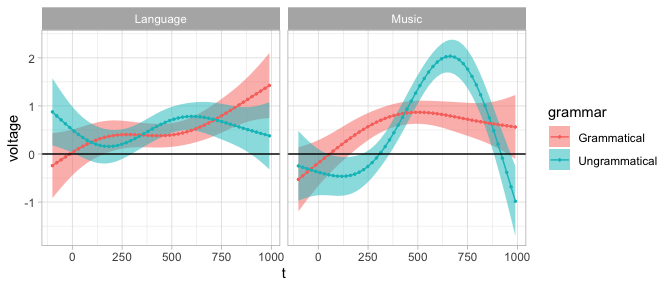If the fs interaction is not removed, the predicted smooth for each individual level in the fs interaction is returned.

predict_gam(
st,
length_out = 50,
series = "t",
separate = list(stim_gram = c("stimulus", "grammar"))
) %>%
plot(comparison = "grammar") +
geom_hline(yintercept = 0) +
facet_grid(~ stimulus)